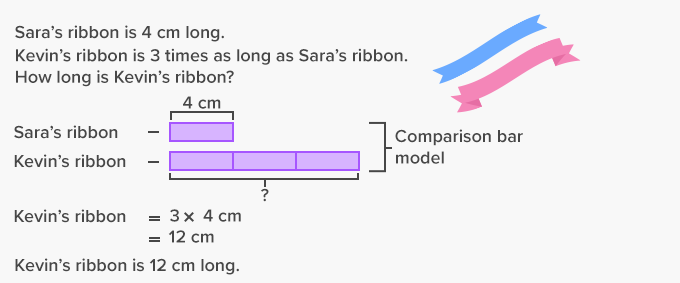# Multiplicative Comparison - Definition with Examples

The Complete K-5 Math Learning Program Built for Your Child

• 40 Million Kids

Loved by kids and parent worldwide

• 50,000 Schools

Trusted by teachers across schools

• Comprehensive Curriculum

Aligned to Common Core

What is Multiplicative Comparison?

Multiplicative comparison means comparing two things or sets that need multiplication..

For example, Sam has twice as many balloons as Sid has.Sid as 3 balloonsSam has twice as many balloons as Sid has. So, number of balloons with Sam = 2 × 3 = 6
Problems for multiplicative comparison are generally word problems and can be solved forming an equation.

A multiplicative comparison problem can be defined as a problem in which:

1. Two verbal statements are used to compare two set of items, and

2. It determines a multiplication equation.

3. The equation includes numbers and alphabet, in which the alphabet is the variable

Example of a multiplicative comparison problem using the equation:

A building is 18 feet tall and the building is three times Jerry’s height. How tall is Jerry?

Let Jerry’s height be “h”

Height of the building = 18 ft which is 3 times Jerry’s height, that is, 3 times h

Therefore, 18 = 3 × h

Dividing both sides by 3, we get:

18 ÷ 3 = 3 × h ÷ 3

6 = h

h = 6 ft

Hence Jerry is 6 ft tall.

Multiplicative comparison problems usually use phrases like:

• Times as many (3 times as many candies)
• Times more (5 times more apples)
• Times as much (7 times as much as the blue ribbon)
• Times as large (10 times as large as a brick)

Here’s an example of how a multiplicative comparison word problem can be solved using comparison bar models.Fun Facts Bar models help us to understand what operations, whether addition, subtraction, multiplication or/and division should be used to solve a word problem. Any two factors and their product can be read as a comparison. In a multiplicative comparison problem, one quantity will always be smaller or larger than the other quantity.

Won Numerous Awards & Honors